# Primary 6 PSLE Practice #4

Time elapsed:
Q1. How many whole numbers from 29 to 62 are divisible by 5?     [1 mark]
Q2. At a fruit stall, the price of 3 mangoes is the same as the price of 5 grapefruits. The price of 3 mangoes is also the same as the price of 10 pears. What is the ratio of the price of a mango to the price of a grapefruit to the price of a pear?     [2 marks]
Q3. Find the value of 14 x 5 - 4 x 4 + 20 - 16     [1 mark]
Q4. The ticketing office at a cinema opens at 10 a.m. The line graph below shows the number of unsold tickets at the ticketing office after three hours. How many ticket were sold in the first hour?     [1 mark]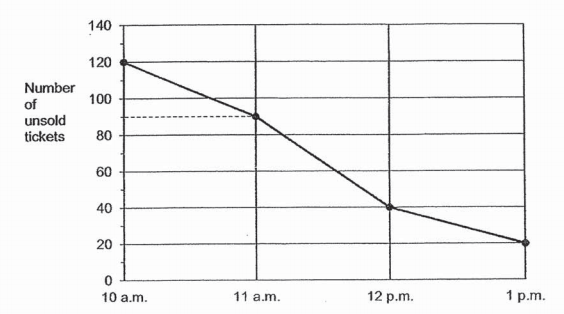Q5. There are 32 apples in a carton. 24 of them are green while the rest are red. What is the ratio of the number of red apples to that of green apples in the carton?     [1 mark]
Q6. Which of the following figures is not symmetrical?     [1 mark]
Q7. The map below shows the locations of 6 places, A, B, C, D, E and F. ABEF and BCDE are squares. Location C is south of location E. Which of the following location is north-east of B?     [2 marks]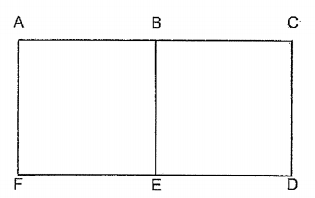Q8. Plank A is 1.5 m longer than Plank B. Plank A is 60 cm longer than Plank C. The total length of the three planks is 12.6 m. Find the length of Plank B.     [2 marks]
Q9. The length of a double-decker bus in Singapore is about     [1 mark]
Q10. In the figure below, ABC is an equilateral triangle. AE and BD are straight lines. Find ∠f.     [2 marks]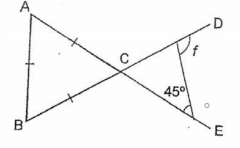Q11. Olivia bought a bottle containing 2.85 ℓ of washing detergent. She used 40 ㎖ of washing detergent each day from Monday to Friday. On Saturday and Sunday, she used 50 ㎖ of washing detergent each day. If Olivia starts using a new bottle on Tuesday, on which day would she use up all the washing detergent?     [2 marks]
Q12. Find the value of 7e - 3 + 2e when e = 4.     [1 mark]
Q13. 1 + 110 + 11000 = ___.     [1 mark]
Q14. The value of the digit 4 in 64 798 is ______.     [1 mark]
Q15. What is the missing number in the box?     [2 marks]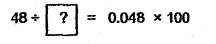Q16. An equilateral triangle and a rectangle have the same perimeter. The length of the rectangle is twice its breadth. The side of the triangle is 12cm. What is the area of the rectangle?     [2 marks]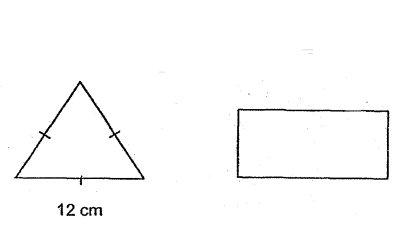Q17. Express 915 as decimal.     [1 mark]
Q18. Which of the following is the same as 6080 g?     [1 mark]
Q19. Express 6 912 as a decimal.     [1 mark]
Q20. Isabelle bought some sugar. She used 13 of the sugar on Monday and 25 of it on Tuesday. The amount of sugar she had left was 132 g. How much sugar did Isabelle buy?     [2 marks]